Chapter 10, Problem 85CP### Introductory Chemistry: A Foundati...

9th Edition
Steven S. Zumdahl + 1 other
ISBN: 9781337399425

#### Solutions

Chapter
Section### Introductory Chemistry: A Foundati...

9th Edition
Steven S. Zumdahl + 1 other
ISBN: 9781337399425
Textbook Problem
1 views

# Which of the following reactions is/are endothermic?l type='a'>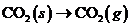i>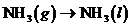i>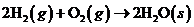i>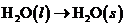i>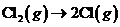Interpretation Introduction

Interpretation:

Which among the given reactions is/are endothermic should be explained.

Concept Introduction:

An exothermic process is the one in which heat energy is produced whereas endothermic is process in which heat energy is absorbed.

Explanation

Reason for correct option:

In an endothermic process heat must be absorbed. Conversion of solid ClCl

CO2 to gaseous CO2 is a process known as sublimation. This needs heat energy

### Still sussing out bartleby?

Check out a sample textbook solution.

See a sample solution

#### The Solution to Your Study Problems

Bartleby provides explanations to thousands of textbook problems written by our experts, many with advanced degrees!

Get Started

#### Find more solutions based on key concepts